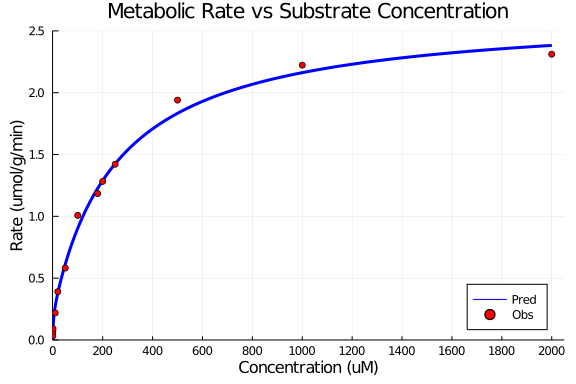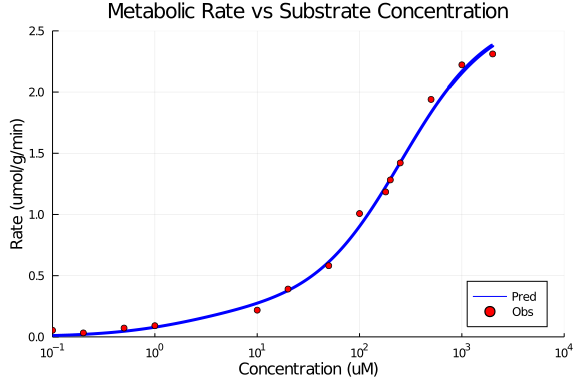# Exercise PK37 - In vitro/In vivo Extrapolation I

### Background

• This exercise aims to fit rate equation to Invitro data

### Learning Outcome

In this exercise, you will learn to assess the relationship between rate and concentration using a non-linear clearance model. The Clearance is impacted by two parallel non-linear processes and both of them are taken into account for calculating rates. The use of a single enzyme system was not able to account for the deviations in the system.

### Libraries

call the "necessary" libraries to get start.

using Pumas
using Plots
using CSV
using StatsPlots
using Random


### Model

pk_37       = @model begin
@param begin
tvvmax1 ∈ RealDomain(lower=0)
tvkm1   ∈ RealDomain(lower=0)
tvvmax2 ∈ RealDomain(lower=0)
tvkm2   ∈ RealDomain(lower=0)
Ω       ∈ PDiagDomain(4)
σ_add   ∈ RealDomain(lower=0)
end

@random begin
η       ~ MvNormal(Ω)
end

@covariates Conc

@pre begin
Vmax1   = tvvmax1 * exp(η)
Km1     = tvkm1 * exp(η)
Vmax2   = tvvmax2 * exp(η)
Km2     = tvkm2 * exp(η)
_Conc   = Conc
end

@derived begin
rate    = @. ((Vmax1*_Conc/(Km1+_Conc)) + (Vmax2*_Conc/(Km2+_Conc)))
dv_rate ~ @. Normal(rate, σ_add)
end
end

PumasModel
Parameters: tvvmax1, tvkm1, tvvmax2, tvkm2, Ω, σ_add
Random effects: η
Covariates: Conc
Dynamical variables:
Derived: rate, dv_rate
Observed: rate, dv_rate


### Parameters

The parameters are as given below. tv represents the typical value for parameters.

• tvvmax1 - Maximum Rate of System 1 (μmol/min/g)

• tvkm1 - Michaelis-Menten constant for system 1 (μmol/L)

• tvvmax2 - Maximum Rate of System 2 (μmol/min/g)

• tvkm2 - Michaelis-Menten constant for system 2 (μmol/L)

param = ( tvvmax1 = 2.43386,
tvkm1   = 256.384,
tvvmax2 = 0.22523,
tvkm2   = 2.23625,
Ω       = Diagonal([0.0,0.0,0.0,0.0]),
σ_add   = 0.0441543)

(tvvmax1 = 2.43386, tvkm1 = 256.384, tvvmax2 = 0.22523, tvkm2 = 2.23625, Ω
= [0.0 0.0 0.0 0.0; 0.0 0.0 0.0 0.0; 0.0 0.0 0.0 0.0; 0.0 0.0 0.0 0.0], σ_a
dd = 0.0441543)


### Dosage Regimen

Since there is no dosage regimen we will create a dataframe with no events and read the file using read_pumas which will be used for simulation.

df_sub1 = DataFrame(id=1, time=0:1:40000 , Conc=0.00:0.05:2000, dv_rate=missing)
sub1    = read_pumas(df_sub1, observations=[:dv_rate], covariates=[:Conc], event_data=false)

Population
Subjects: 1
Covariates: Conc
Observables: dv_rate


### Simulations

We willnow simulate the rate using the simobs function

Random.seed!(123)
sim_sub1 = simobs(pk_37, sub1, param)
df1      = DataFrame(sim_sub1)


### Dataframe and Plotting

Use the dataframe for plotting

df1_dv = filter(x -> x.Conc in [0.02,0.05,0.1,0.2,0.5,1,10,20,50,100,180,200,250,500,1000,2000], df1)

@df df1 plot(:Conc, :rate,
title="Metabolic Rate vs Substrate Concentration", xlabel= "Concentration (uM)", ylabel="Rate (umol/g/min)",
label="Pred", linewidth=3, color=[:blue], legend=:bottomright,
xticks=[0,200,400,600,800,1000,1200,1400,1600,1800,2000], xlims=(-1,2050),
yticks=[0,0.5,1.0,1.5,2.0,2.5], ylims=(0,2.5))
@df df1_dv scatter!(:Conc, :dv_rate, label="Obs", color=[:red])@df df1 plot(:Conc, :rate, xaxis=:log,
title="Metabolic Rate vs Substrate Concentration", xlabel= "Concentration (uM)", ylabel="Rate (umol/g/min)",
label="Pred", linewidth=3, color=[:blue], legend=:bottomright,
xticks=[0.01,0.1,1,10,100,1000,10000], xlims=(0.1,10000),
yticks=[0,0.5,1.0,1.5,2.0,2.5], ylims=(0,2.5))
@df df1_dv scatter!(:Conc, :dv_rate, label="Obs", color=[:red])